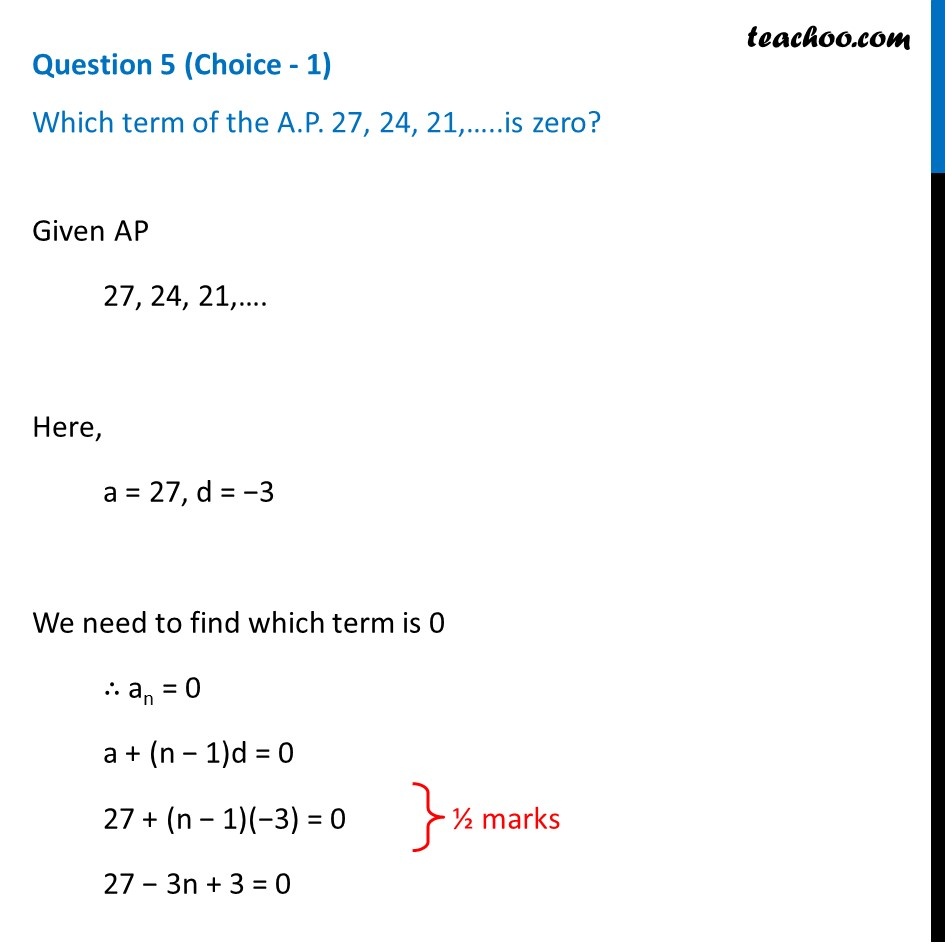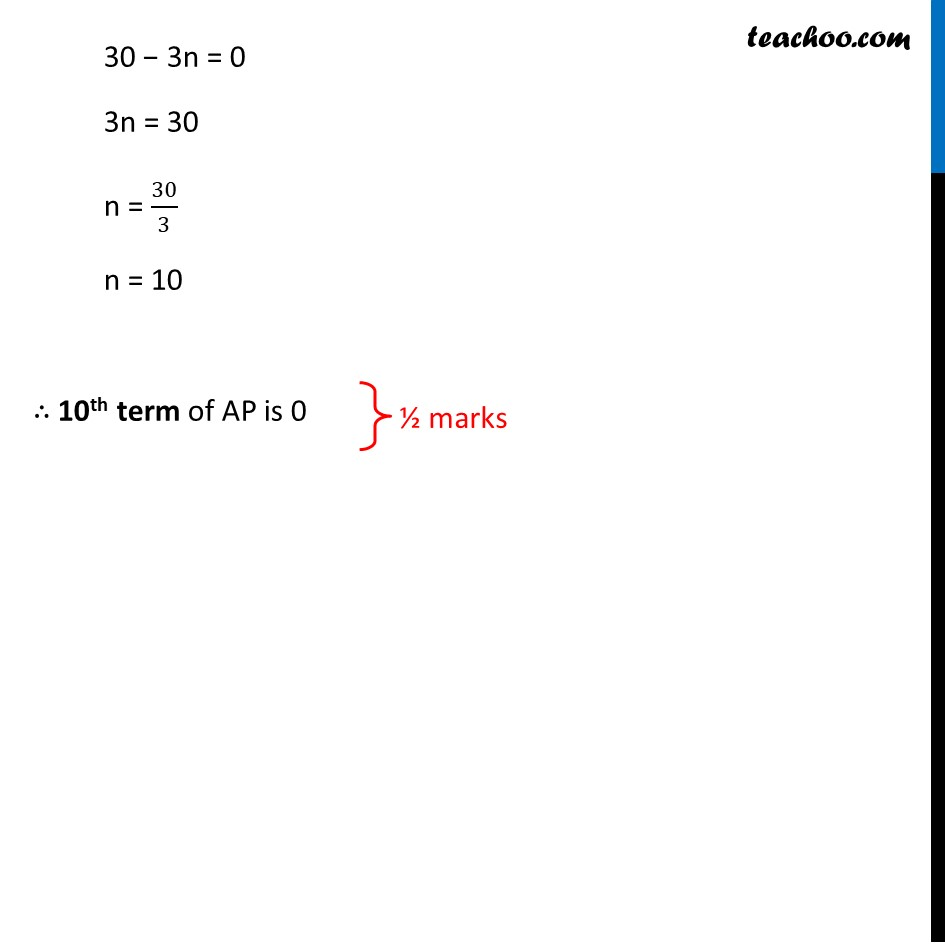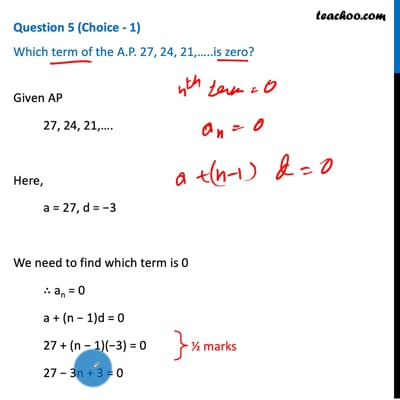CBSE Class 10 Sample Paper for 2021 Boards - Maths Standard

Class 10
Solutions of Sample Papers for Class 10 Boards

## Which term of the A.P. 27, 24, 21,…..is zero?This video is only available for Teachoo black users

Introducing your new favourite teacher - Teachoo Black, at only ₹83 per month

### Transcript

Question 5 (Choice - 1) Which term of the A.P. 27, 24, 21,…..is zero? Given AP 27, 24, 21,…. Here, a = 27, d = −3 We need to find which term is 0 ∴ an = 0 a + (n − 1)d = 0 27 + (n − 1)(−3) = 0 27 − 3n + 3 = 0 30 − 3n = 0 3n = 30 n = 30/3 n = 10 ∴ 10th term of AP is 0